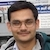# NAEST 2015 Screening Test: Balancing a T Shaped DandaBy

A 'T' shaped danda is made by joining one end of a pipe to the midpoint of another pipe (using a T joint). This danda can be used to explain rotational equilibrium through an entertaining demonstration.

Question 1: The weight of the hanging mass is $W$. The net force applied by the man on the danda is $F_1$ in case 1 and it is $F_2$ in case 2. Which of the following is(are) correct?

1. $F_1=W, F_2 > W$
2. $F_1 = W, F_2 = W$
3. $F_1 = W, F_2 < W$
4. $F_1 \neq W, F_2 \neq W$

Question 2: All torques are about the axis of the pipe in hand. The torque of $F_1$ is $\tau_1$ and that of $F_2$ is $\tau_2$. The torque of $W$ in case 1 is $\tau_3$ and that in case 2 is $\tau_4$. Which of the following is(are) correct?

1. $\tau_1 = \tau_3, \tau_2=\tau_4$
2. $\tau_2 > \tau_1$
3. $\tau_2 = \tau_1$
4. $\tau_2 < \tau_1$

Answer: The danda is kept in the equilibrium state by holding it with hands. The conditions for the equilibrium are,

1. the net force on the danda is zero
2. the net torque on the danda (about any point) is zeroThe forces acting on the danda are (a) its weight $W$ and (b) the force applied by the hands - $F_1$ in case 1 and $F_2$ in case 2. The net force on the danda when it is kept horizontal is zero. Hence, $F_1=W$ and $F_2=W$.

Let the perpendicular distance of the hanging mass from the axis of the pipe be $d_1$ in case 1 and $d_2$ in case 2 (see figure). The torque of $W$ in case 1 and case 2 are given by \begin{align} \tau_3 & =d_1 W, \\ \tau_4 & = d_2 W. \end{align} Since $d_2>d_1$, we get $\tau_4 > \tau_3$.

The net torque on the danda is zero because it is in equilibrium. Thus, $\tau_1=\tau_3$ and $\tau_2=\tau_4$. Note that these torques are equal in magnitude but opposite in directions. The condition $\tau_4 > \tau_3$ gives $\tau_2 > \tau_1$.

Let us extend this discussion a little further. Let the radius of the pipe in hands be $r$. The hands apply tangential frictional force at each point on the periphery of the danda.The frictional force by the hand on the danda is equivalent to

1. force $f_A$ at point A (downward)
2. force $f_B$ at point B (upward)

The equilibrium condition gives \begin{align} &f_B=f_A+W, \\ &f_A r +f_B r=W d. \end{align} Solve to get \begin{align} f_A&=\frac{W}{2}\left(\frac{d}{r}-1\right), \\ f_B&=\frac{W}{2}\left(\frac{d}{r}+1\right). \end{align} Note that the net force applied by the hands, $F=f_B-f_A=W$, is independent of $d$. However, forces $f_A$ and $f_B$ depends on $d$. Their magnitudes are much greater than $W$ because $d \gg r$.

I encourage you to hold one end of a pencil between the thumb and the forefinger of the left hand (see figure). Observe the direction of forces at the thumb and the forefinger when the pencil is horizontal. What happens when you push down the free end (tip) of the pencil with your right hand?